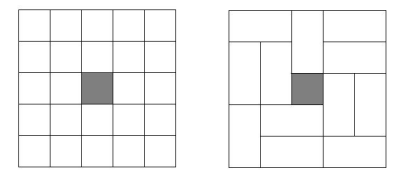# 紫色彗星数学竞赛真题（2016年初中组）

PURPLE COMET! MATH MEET April 2016
MIDDLE SCHOOL - PROBLEMS

Problem 1
Mike has 12 books, Sean has 9 books, and little Sherry has only 4 books. Find the percentage of these books that Sean has.
Problem 2
The figure below was formed by taking four squares, each with side length 5, and putting one on each side of a square with side length 20. Find the perimeter of the figure below.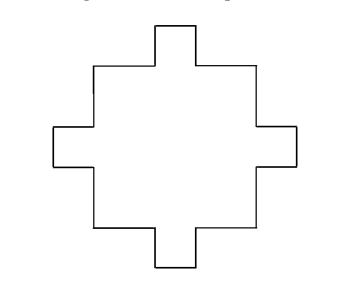Problem 3
The sum of the numbers 3a − 4, 3b − 4, and 3c − 4 is 2016. Find the sum of the numbers 4a − 3, 4b− 3,and 4c − 3.
Problem 4
The following diagram shows a square where each side has four dots that divide the side into three equal segments. The shaded region has area 105. Find the area of the original square.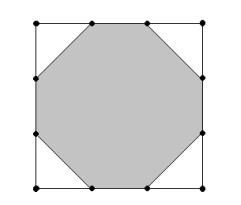Problem 5
A 2 meter long bookshelf is filled end-to-end with 46 books. Some of the books are 3 centimeters thick while all the others are 5 centimeters thick. Find the number of books on the shelf that are 3 centimeters thick.
Problem 6
Find the number of three-digit positive integers where the digits are three different prime numbers. For example, count 235 but not 553.
Problem 7
Melanie has 4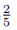cups of flour. The recipe for one batch of cookies calls for 1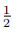cups of flour. Melanie plans to make 2batches of cookies. When she is done, she will have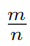cups of flour remaining, where m and n are relatively prime positive integers. Find m + n.
Problem 8
The figure below has a 1 × 1 square, a 2 × 2 square, a 3 × 3 square, a 4 × 4 square, and a 5 × 5 square. Each of the larger squares shares a corner with the 1 × 1 square. Find the area of the region covered by these five squares.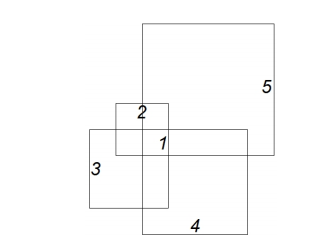Problem 9
Find the value of x such that 2x+3 − 2x−3 = 2016.
Problem 10
Mildred the cow is tied with a rope to the side of a square shed with side length 10 meters. The rope is attached to the shed at a point two meters from one corner of the shed. The rope is 14 meters long. The area of grass growing around the shed that Mildred can reach is given by nπ square meters, where n is a positive integer. Find n.
Problem 11
One evening a theater sold 300 tickets for a concert. Each ticket sold for \$40, and all tickets were purchased using \$5, \$10, and \$20 bills. At the end of the evening the theater had received twice as many \$10 bills as \$20 bills, and 20 more \$5 bills than \$10 bills. How many bills did the theater receive altogether?
Problem 12
Find the number of squares such that the sides of the square are segments in the following diagram and where the dot is inside the square.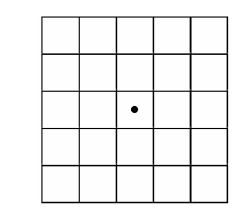Problem 13
One afternoon Elizabeth noticed that twice as many cars on the expressway carried only a driver as compared to the number of cars that carried a driver and one passenger. She also noted that twice as many cars carried a driver and one passenger as those that carried a driver and two passengers. Only 10% of the cars carried a driver and three passengers, and no car carried more than four people. Any car containing at least three people was allowed to use the fast lane. Elizabeth calculated thatof the people in cars on the expressway were allowed to ride in the fast lane, where m and n are relatively prime positive integers. Find m + n.
Problem 14
Find the number of positive integers n such that a regular polygon with n sides has internal angles with measures equal to an integer number of degrees.
Problem 15
The real numbers x, y, and z satisfy the system of equations
x2 + 27 = −8y + 10z
y2 + 196 = 18z + 13x
z2 + 119 = −3x + 30y.
Find x + 3y + 5z.
Problem 16
The figure below shows a barn in the shape of two congruent pentagonal prisms that intersect at right angles and have a common center. The ends of the prisms are made of a 12 foot by 7 foot rectangle surmounted by an isosceles triangle with sides 10 feet, 10 feet, and 12 feet. Each prism is 30 feet long. Find the volume of the barn in cubic feet.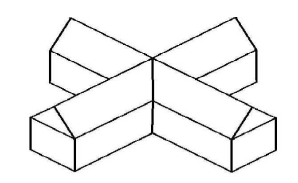Problem 17
Suzie flips a fair coin 6 times. The probability that Suzie flips 3 heads in a row but not 4 heads in a row is given by, where m and n are relatively prime positive integers. Find m + n.
Problem 18
Find the least positive integer N that is 50 times the number of positive integer divisors that N has.
Problem 19
Find the positive integer n such that the least common multiple of n and n − 30 is n + 1320.
Problem 20
The 24 unshaded squares in the 5 × 5 grid below can be tiled with twelve 1 × 2 tiles. One such tiling is shown. Find the number of ways the grid can be tiled.